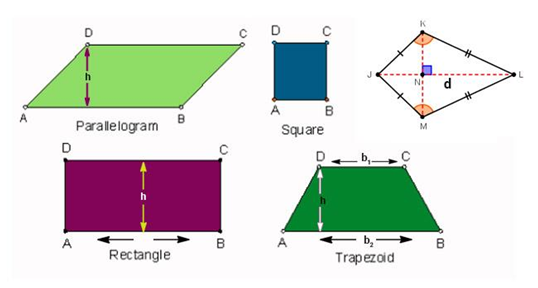A quadrilateral is a closed figure that has four sides in it. The interior angles add up to 360 degrees. Also, for some quadrilaterals, the opposite sides are parallel and opposite angles are equal. Quadrilaterals can be classified into different types some of them are square, rectangle, parallelogram, rhombus and trapezoid. Five different formulas are used to calculate the area of the quadrilateral.

In parallelogram adjacent sides are of unequal lengths and angles are oblique (not right angles). In a rectangle, all four angles are right angles. An equivalent condition is that the diagonals bisect each other and are equal in length.Area of a Square (side)2
Area of a Kite (1 ⁄ 2) × Product of Diagonals
Area of a Parallelogram Base × Height
Area of a Rectangle Length × Breadth
Area of a Trapezoid
$$\begin{array}{l}\frac{base_{1}\,+\,base_{2}}{2}\times height\end{array}$$

### Solved Example For Quadrilateral Formula for Area

Question: Find the area of a trapezoid with bases of 11 inches and 12 inches, and a height of 5 inches.

Solution:

Given, b1= 11 inches

b2 = 12 inches

h = 5 inches

Area of Trapezoid =

$$\begin{array}{l}\frac{base_{1}+base_{2}}{2}\times Hieght = \frac{11+12}{2} \times 5\end{array}$$

=

$$\begin{array}{l}\frac{23}{2}\times 5\end{array}$$

= 57.5 in2